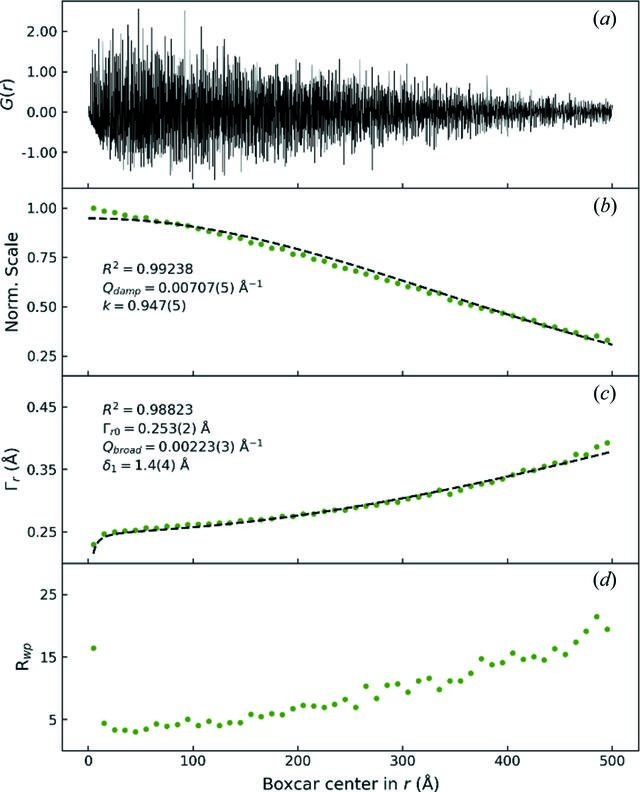disable zoom     view article Figure 4 (a) The PDF of Si at 300 K with a range of r = 500 Å based on the total-scattering data collected with OHGI. (b)–(d) Boxcar-refinement results of the PDF for normalized scale factors (b), the refined FWHM Γr (c) and Rwp values (d). The refined values are shown by green dots. The Γr values were calculated using the equation Γr = 2[2 ln(2)]1/2(U1 + U2)1/2, where U1 and U2 are the ADPs of the two atoms separated by the interatomic distance r. In the case of Si, U1 = U2 = USi, which leads to. The dashed lines in (b) and (c) represent least-squares fits with the correction functions for PDF damping and peak broadening given by Qdamp and Qbroad, respectively. R2 denotes the correlation coefficient of the fit and k in (b) is the intercept with the vertical axis.

IUCrJ
Volume 8| Part 3| May 2021| Pages 387-394
ISSN: 2052-2525